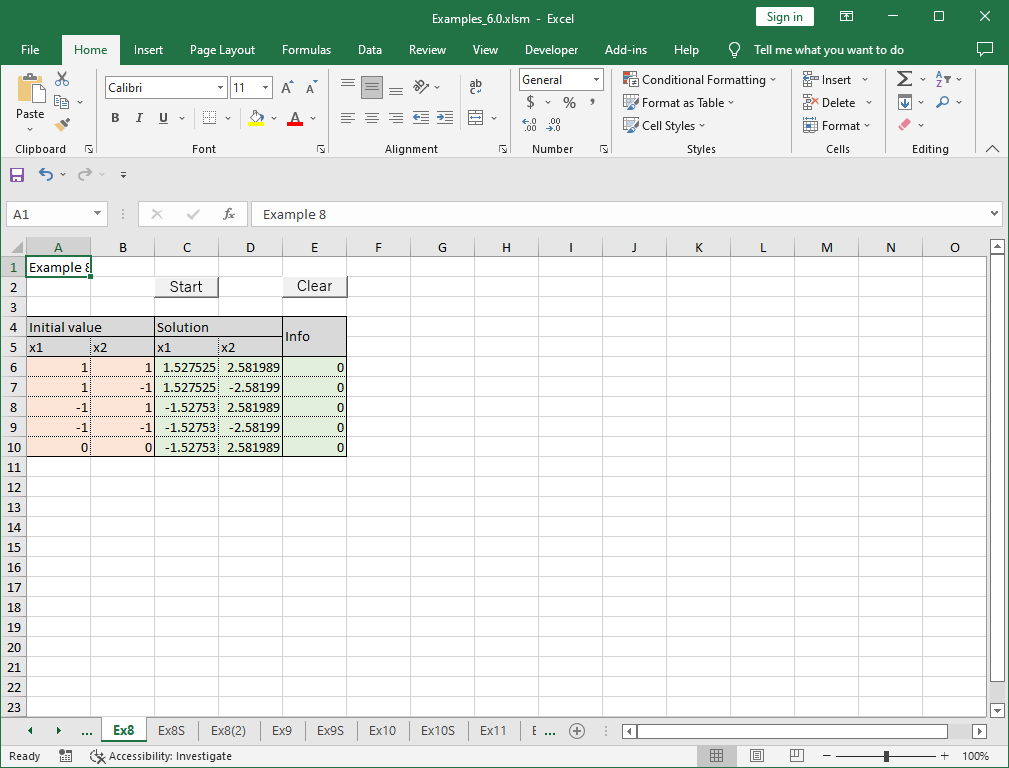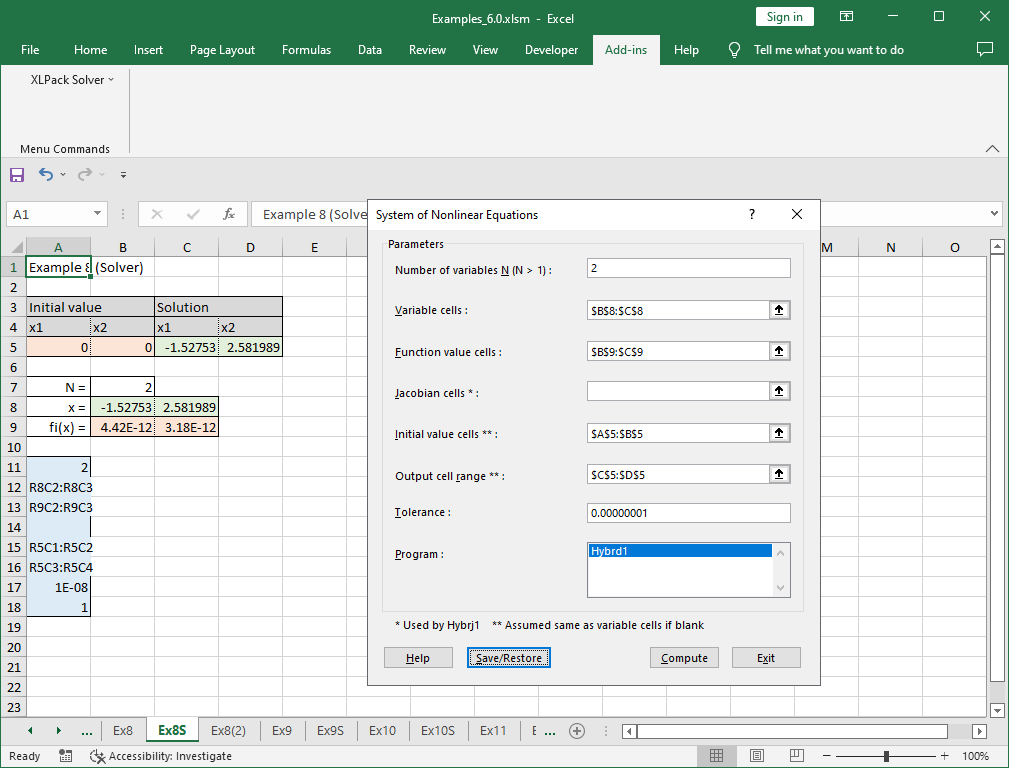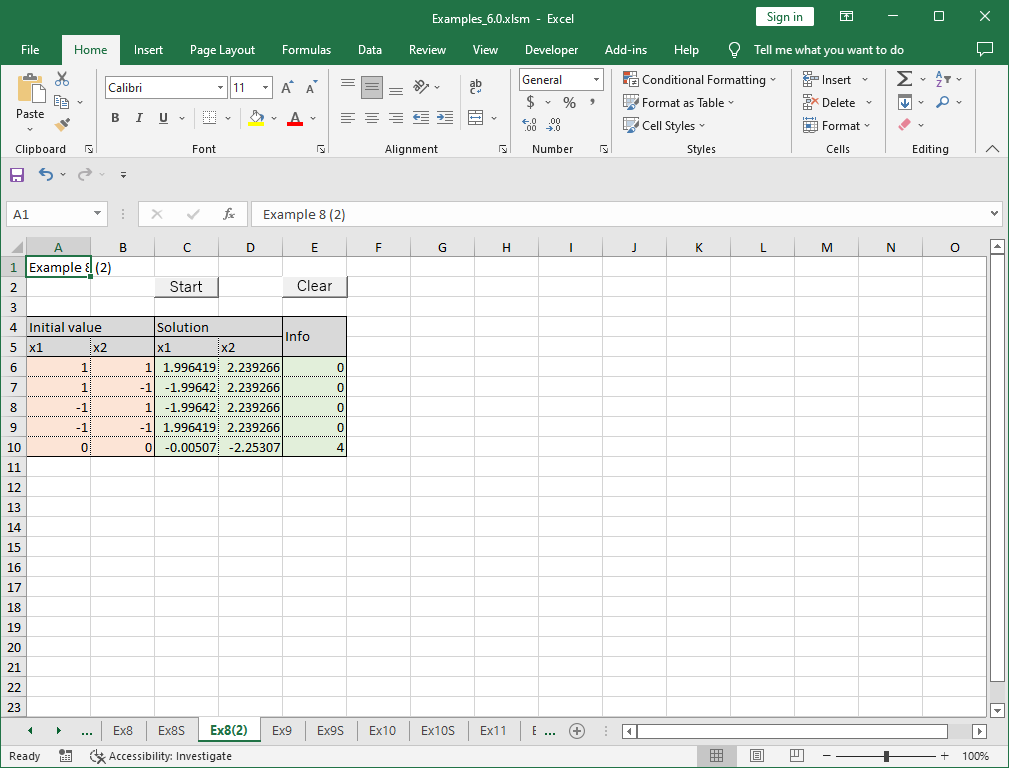# 8. System of nonlinear equations

Note – The example worksheets are available for download. See 1. How to obtain and use example worksheets.

Examples of obtaining real solutions of a system of general nonlinear equations by XLPack are shown below.

### Example 1

Let’s solve the following system of nonlinear equations.

```
f1(x1, x2) = 4x12 + x22 - 16 = 0
f2(x1, x2) = x12 + x22 - 9 = 0

```

The plot of these equations is as follows.We see there are four real solutions symmetrically about (0, 0).

### Solving problem with VBA program (1)

The following is an example code using VBA library subroutine Hybrd1.

``````Sub F(N As Long, X() As Double, Fvec() As Double, IFlag As Long)
Fvec(0) = 4 * X(0) ^ 2 + X(1) ^ 2 - 16
Fvec(1) = X(0) ^ 2 + X(1) ^ 2 - 9
End Sub

Sub Start()
Const NMax = 10
Dim N As Long, X(NMax) As Double, Fvec(NMax) As Double, XTol As Double
Dim Info As Long, I As Long
'--- Initialization
N = 2
XTol = 0.000000000001 '1e-12
For I = 0 To 4
'--- Input data
X(0) = Cells(6 + I, 1): X(1) = Cells(6 + I, 2)
'--- Compute zeros of system of equations
Call Hybrd1(AddressOf F, N, X(), Fvec(), XTol, Info)
'--- Output zeros
Cells(6 + I, 3) = X(0): Cells(6 + I, 4) = X(1): Cells(6 + I, 5) = Info
Next
End Sub``````

You need to define the object function as the external subroutine and call Hybrd1 with specifying the initial value and the tolerance. Hybrd1 starts the iterative calculation from the given initial approximation and find the solution located near. The above program is designed to see what solutions are obtained when five different initial values are given.

In this example, we try four initial approximations (1, 1), (-1, 1), (1, -1) and (-1, -1) aiming to all four solutions. And we also test the center point (0, 0) as the initial approximation.

The following result was obtained by running this program.Each one converged to the nearest solution for four initial approximation cases. When started from (0, 0), it converged to the upper left solution.

### Solving problem with VBA program (2)

The following is an example code using the reverse communication version VBA library subroutine Hybrd1_r.

``````Sub Start()
Const NMax = 10
Dim N As Long, X(NMax) As Double, Fvec(NMax) As Double, XTol As Double
Dim Info As Long, I As Long
Dim XX(NMax - 1) As Double, YY(NMax - 1) As Double, IRev As Long
'--- Initialization
N = 2
XTol = 0.000000000001 '1e-12
For I = 0 To 4
'--- Input data
X(0) = Cells(6 + I, 1): X(1) = Cells(6 + I, 2)
'--- Compute zeros of system of equations
IRev = 0
Do
Call Hybrd1_r(N, X(), Fvec(), XTol, Info, XX(), YY(), IRev)
If IRev = 1 Or IRev = 2 Then
YY(0) = 4 * XX(0) ^ 2 + XX(1) ^ 2 - 16
YY(1) = XX(0) ^ 2 + XX(1) ^ 2 - 9
End If
Loop While IRev <> 0
'--- Output zeros
Cells(6 + I, 3) = X(0): Cells(6 + I, 4) = X(1): Cells(6 + I, 5) = Info
Next
End Sub``````

Instead of defining the object function as the external function, when returned with IRev = 1 or 2, you need to compute the object function values at XX(), enter them in YY() and call Hybrd1_r again. For further details of reverse communication version, see here.

The same result with above is obtained by this program.

### Solving problem with Solver

“System of Nonlinear Equations” of XLPack solver add-in can be used to solve this problem. The formulas (=4*B8^2+C8^2-16 and =B8^2+C8^2-9) are entered in cells B9 and C9.Please refer to here for how to use solver.

### Example 2

Let’s make small change of the equations of example 1 as follows.

```
f1(x1, x2) = 4x12 + (x2 - 2)2 - 16 = 0
f2(x1, x2) = x12 + x22 - 9 = 0

```

The plot of these equations is as follows.Now, there are only two real solutions. The following result was obtained by the VBA program with same initial values as example 1.For the initial values (1, 1) and (-1, 1), it converged to nearest solutions. But for (1, -1) and (-1, -1), it converged unexpectedly to rather far solutions. When started from (0, 0), it failed to converge. We see that the desired solution cannot be obtained if the initial value is not good approximation of the desired solution. It is important to supply the initial approximation as good as possible.